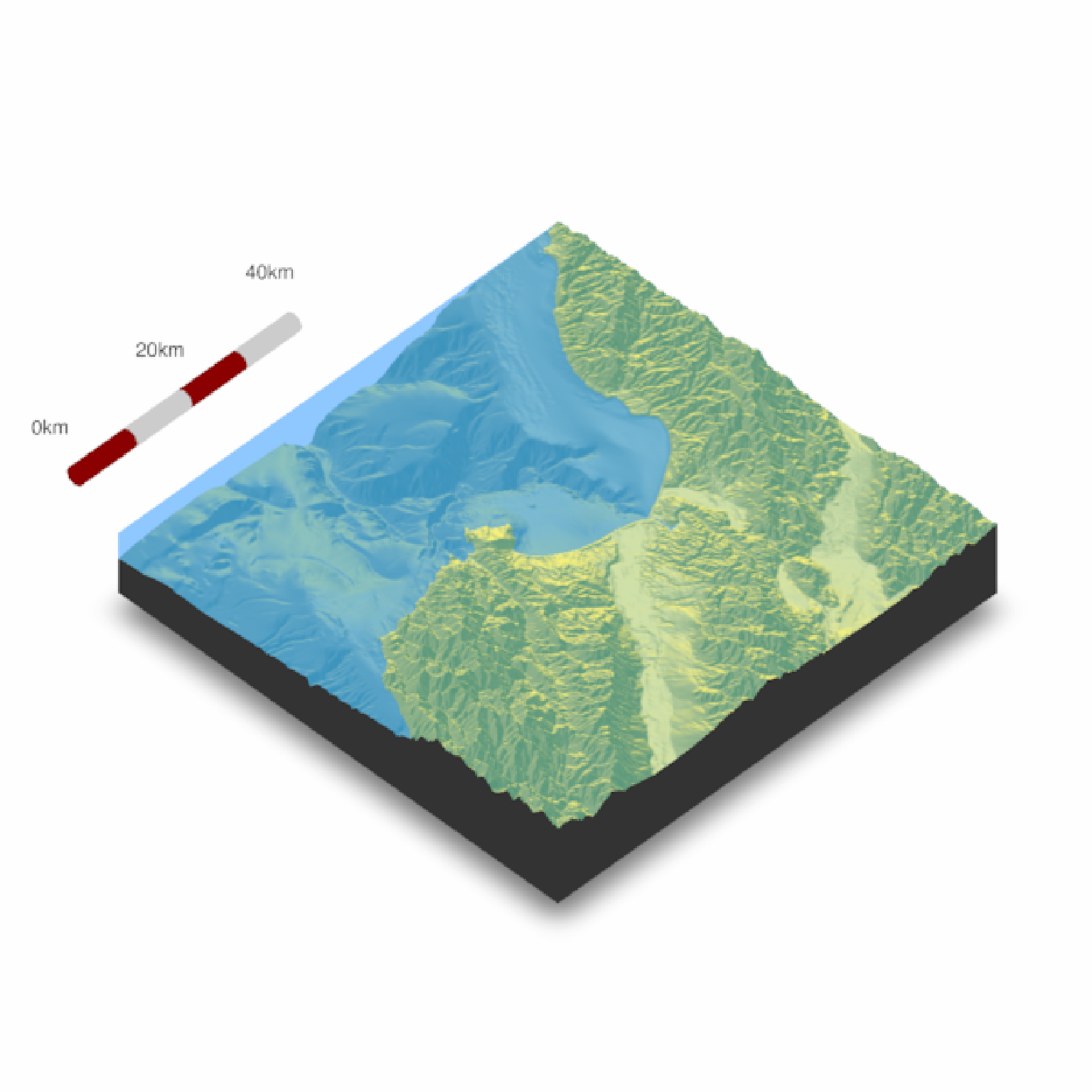Places a scale bar on the map in 3D.

render_scalebar(
limits,
position = "W",
y = NULL,
segments = 10,
scale_length = 1,
label_unit = "",
offset = NULL,
color_first = "darkred",
color_second = "grey80",
color_text = "black",
text_switch_side = FALSE,
text_x_offset = 0,
text_y_offset = 0,
text_z_offset = 0,
clear_scalebar = FALSE
)

## Arguments

limits

The distance represented by the scale bar. If a numeric vector greater than length 1, this will specify the breaks along the scale bar to place labels, with the maximum value in limits assumed to be the last label. Must be non-negative.

position

Default W. A string representing a direction. Can be N, E, S, and W.

y

Default NULL. The height of the scale bar, automatically calculated if NULL.

segments

Default 10. Number of colored segments in the scalebar.

scale_length

Default 1. Length of the scale bar, relative to the side of the map specified in position. If a length-2 vector, the first number specifies the start and stop points along the side.

label_unit

Default NULL. The distance unit for the label.

offset

Default NULL. The distance away from the edge to place the scale bar. If NULL, automatically calculated.

Default NULL. The radius of the cylinder representing the scale bar. If NULL, automatically calculated.

color_first

Default darkred. Primary color in the scale bar.

color_second

Default grey90. Seconary color in the scale bar.

color_text

Default black. Color of the text.

text_switch_side

Default FALSE. Switches the order of the text.

text_x_offset

Default 0. Distance offset for text in the x direction.

text_y_offset

Default 0. Distance offset for text in the y direction.

text_z_offset

Default 0. Distance offset for text in the z direction.

clear_scalebar

Default FALSE. Clears the scale bar(s) on the map.

## Value

Displays snapshot of current rgl plot (or saves to disk).

## Examples

#Add a scale bar to the montereybay dataset, here representing about 80km
if(run_documentation()) {
montereybay %>%
plot_3d(montereybay,theta=45, water=TRUE)
render_scalebar(limits=c(0, 80), label_unit = "km")
render_snapshot()
}
#> montereybay dataset used with no zscale--setting zscale=50.  For a realistic depiction, raise zscale to 200.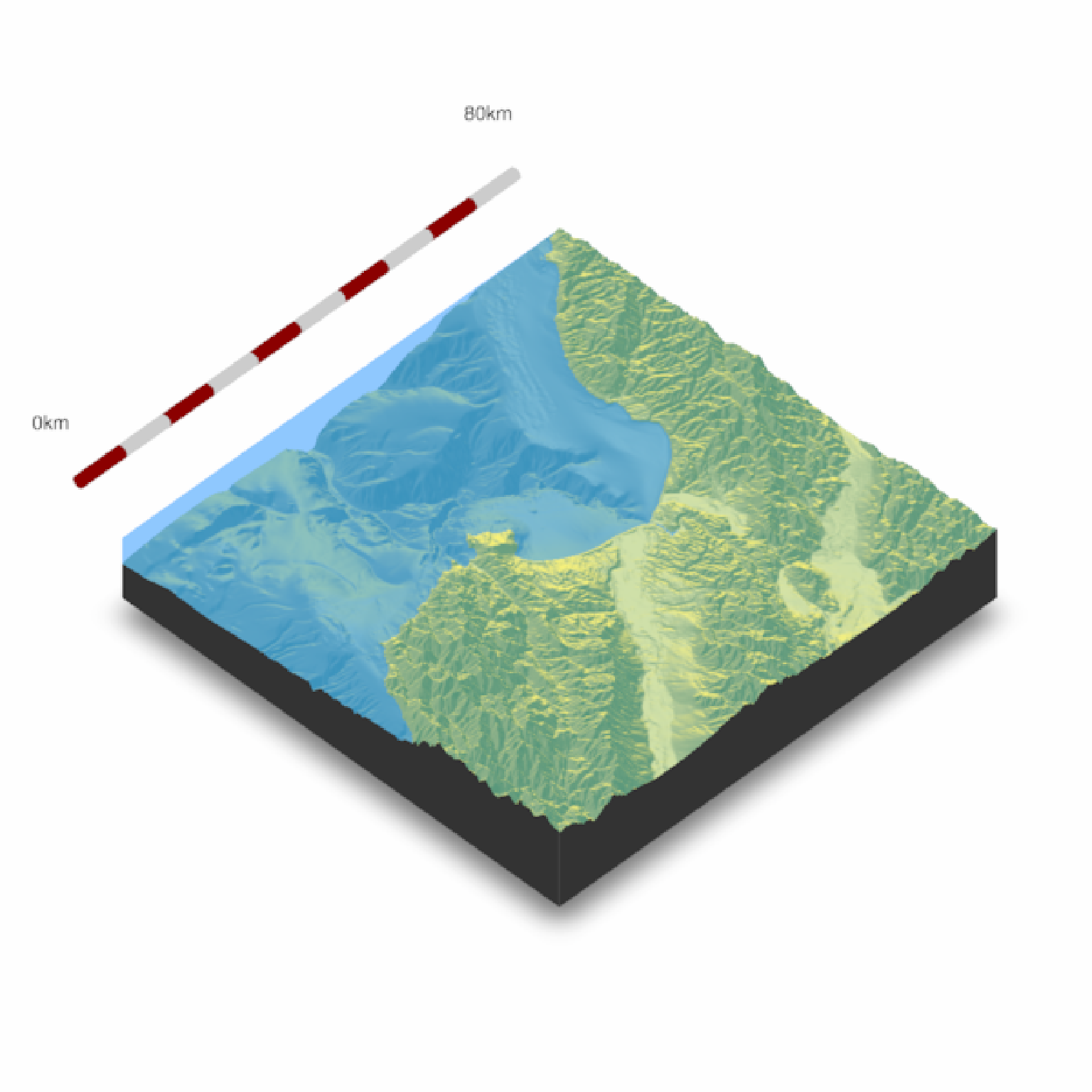if(run_documentation()) {
#This function works with render_highquality()
render_highquality(lightdirection = 250, lightaltitude = 40,
scale_text_size = 24, clamp_value = 10,
sample_method = "sobol_blue", samples = 128)
render_scalebar(clear_scalebar = TRUE)
}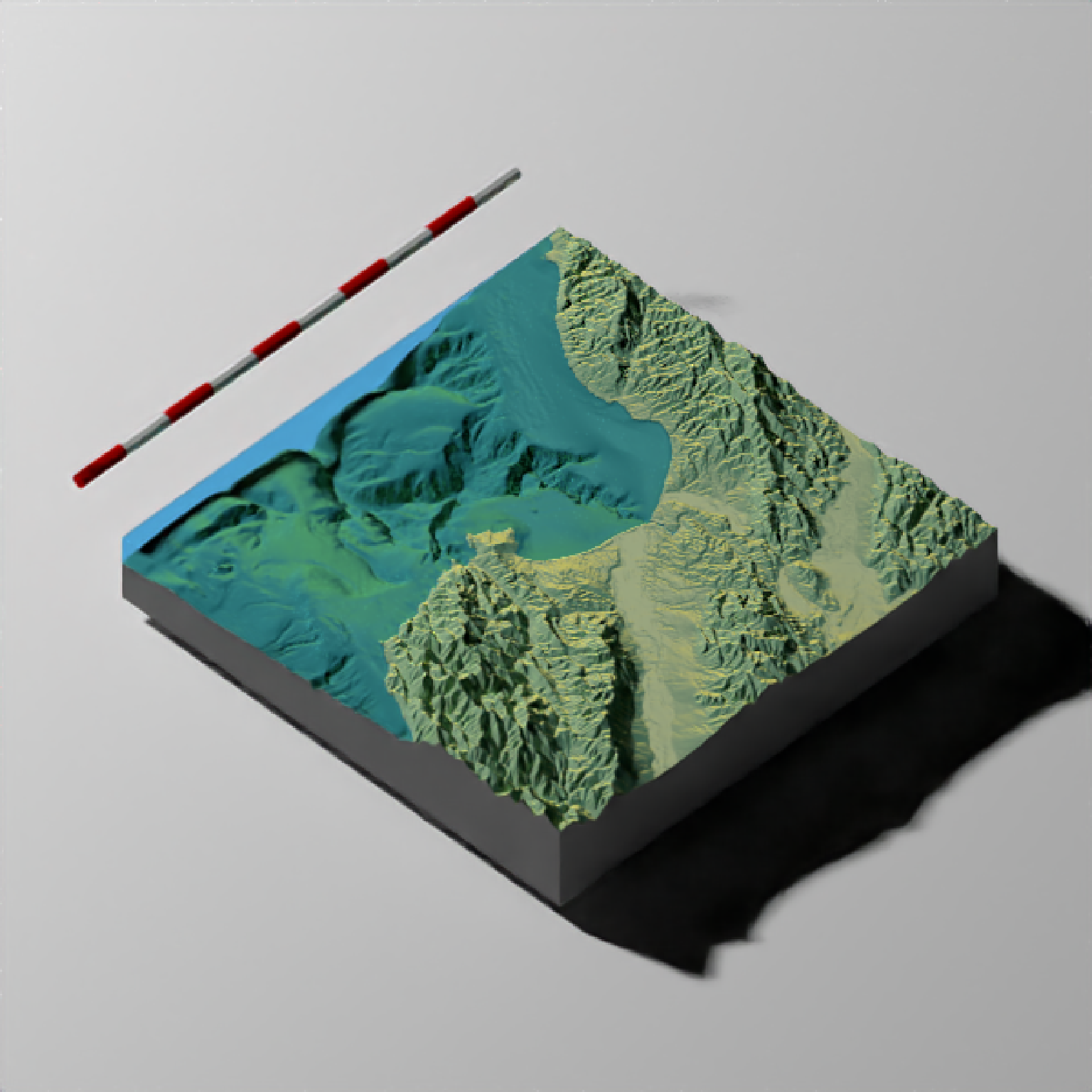if(run_documentation()) {
#We can change the position by specifying a cardinal direction to position, and the
#color by setting color_first and color_second

render_scalebar(limits=c(0,80), label_unit = "km", position = "N",
color_first = "darkgreen", color_second = "lightgreen")
render_snapshot()
render_scalebar(clear_scalebar = TRUE)
}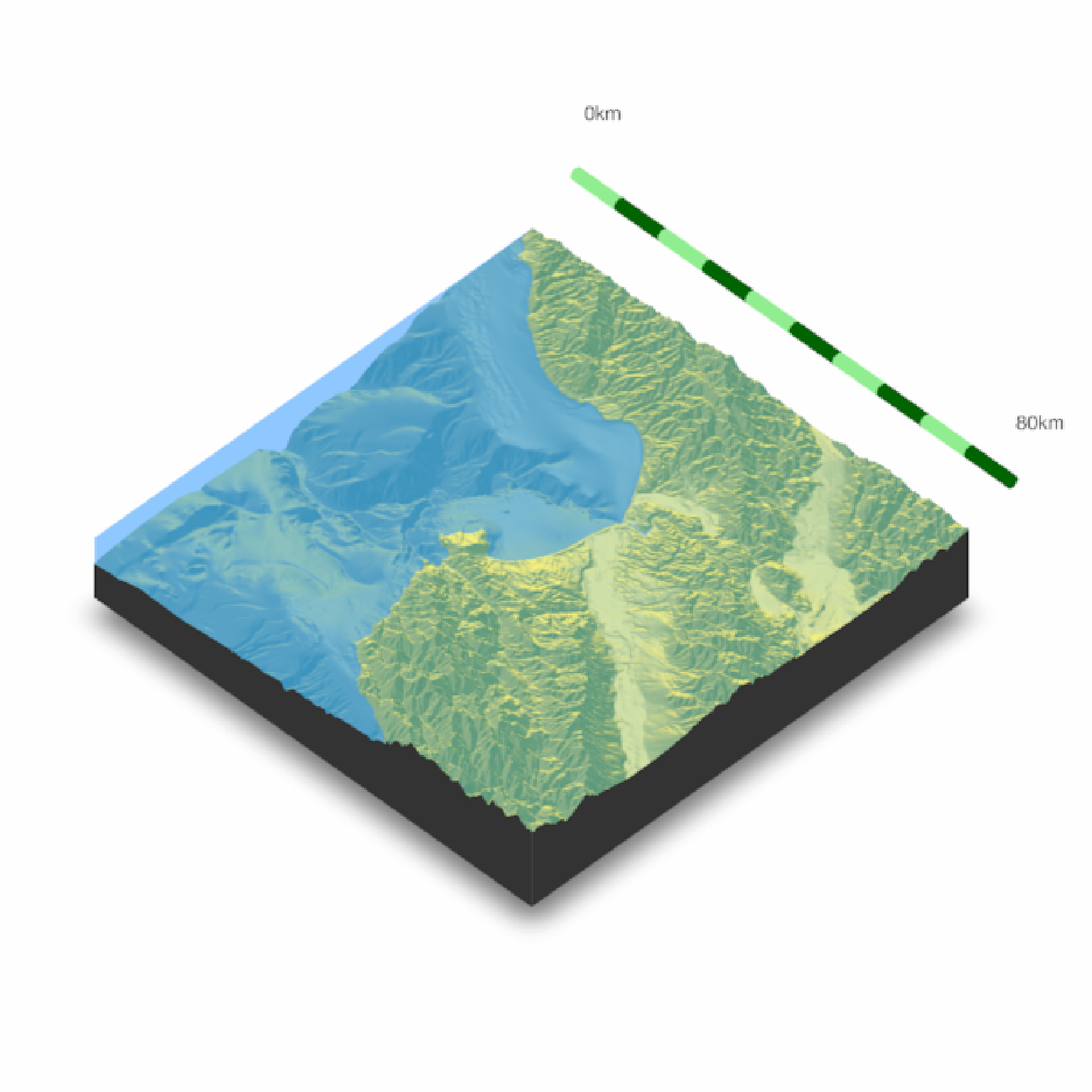if(run_documentation()) {
#And switch the orientation by setting text_switch_side = TRUE
render_scalebar(limits=c(0,80), label_unit = "km", position = "N", text_switch_side = TRUE,
color_first = "darkgreen", color_second = "lightgreen")
render_snapshot()
render_scalebar(clear_scalebar = TRUE)
}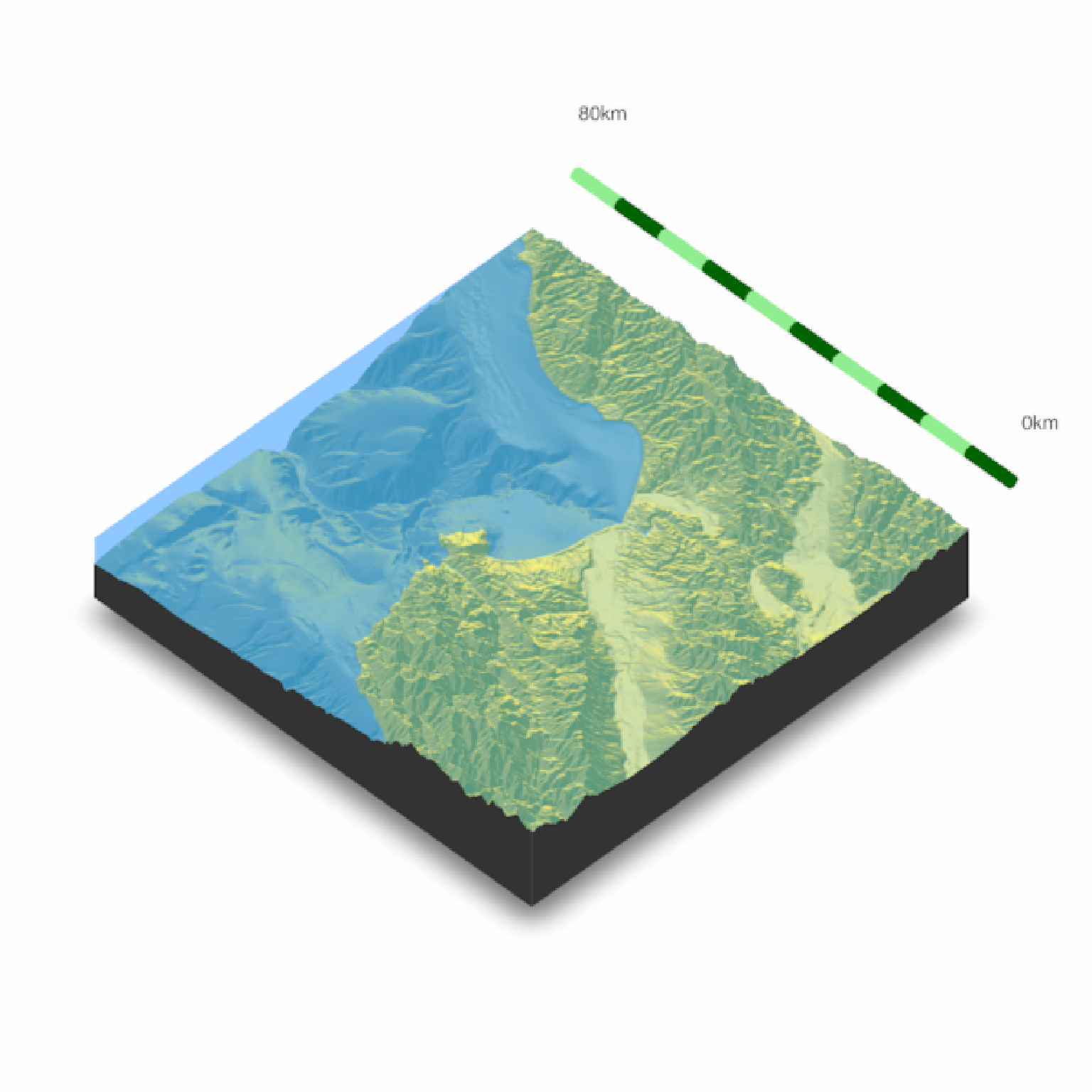if(run_documentation()) {
#We can add additional breaks by specifying additional distances in limits

render_scalebar(limits=c(0,40,80), label_unit = "km")
render_snapshot()
render_scalebar(clear_scalebar = TRUE)
}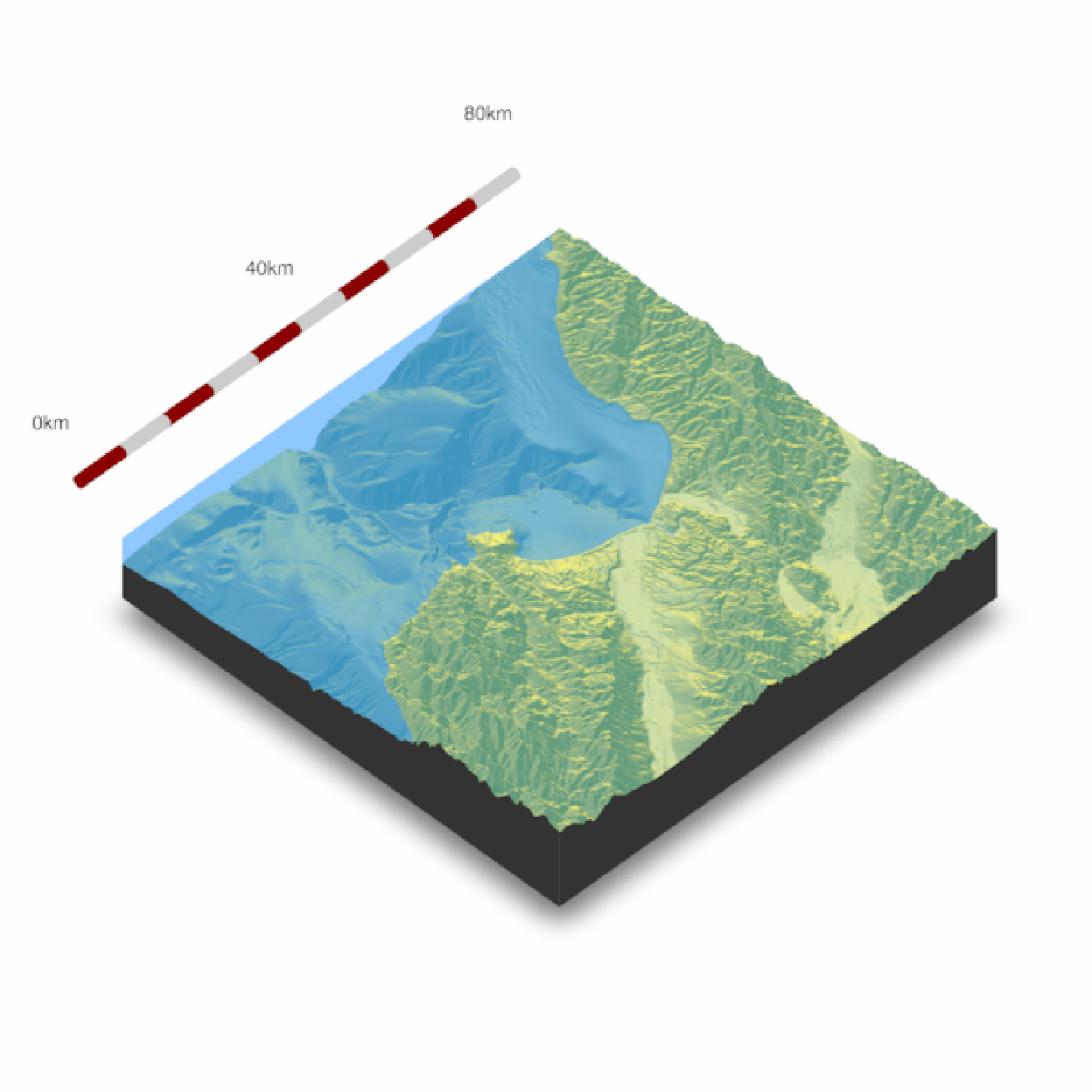if(run_documentation()) {
#We can also manually specify the height by setting the y argument:

render_scalebar(limits=c(0,40,80), y=-70, label_unit = "km")
render_snapshot()
render_scalebar(clear_scalebar = TRUE)
}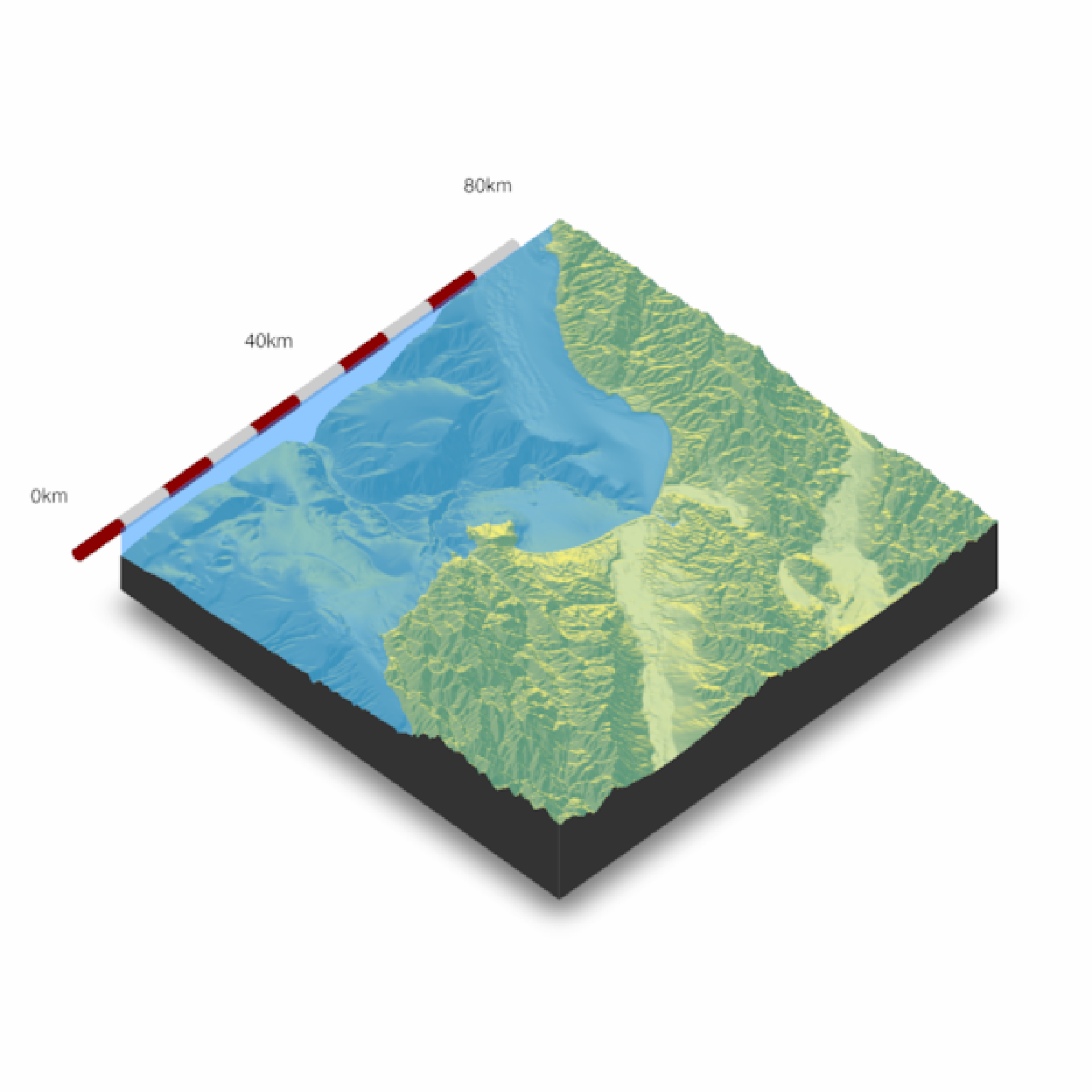if(run_documentation()) {
#Here we change the total size by specifying a start and end point along the side,
#and set the number of colored segments:

render_scalebar(limits=c(0,20, 40), segments = 4, scale_length = c(0.5,1), label_unit = "km")
render_scalebar(limits=c(0,20, 40), segments = 4, position = "N", text_switch_side = TRUE,
scale_length = c(0.25,0.75), label_unit = "km")
render_snapshot()
render_scalebar(clear_scalebar = TRUE)
}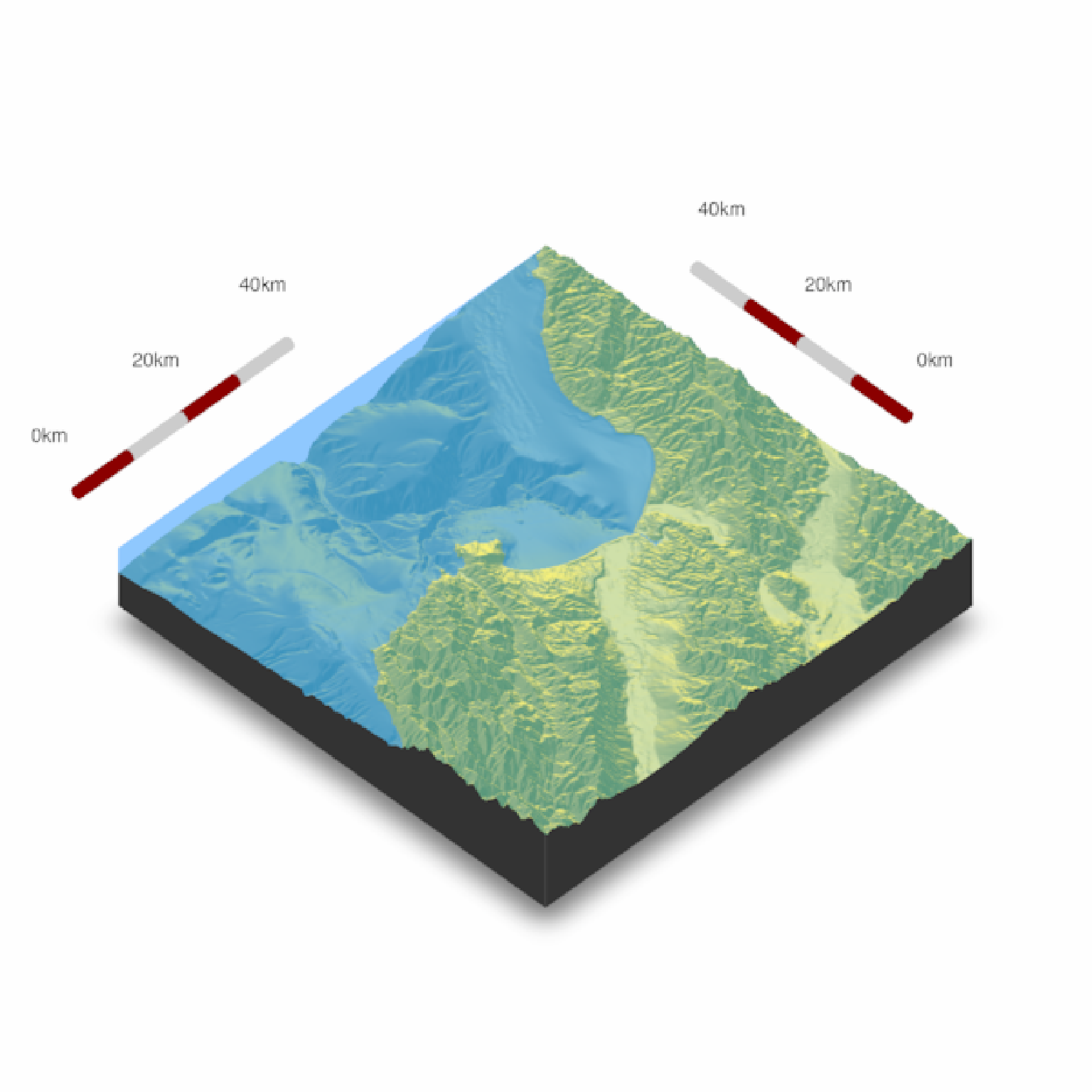if(run_documentation()) {
#Change the radius of the scale bar with radius. Here, the autopositioning doesn't work well with
#the labels, so we provide additional offsets with text_y_offset and text_x_offset to fix it.

render_scalebar(limits=c(0,20, 40), segments = 4, scale_length = c(0.5,1),